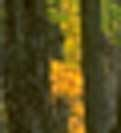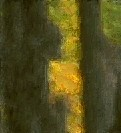# CS129 FINAL PROJECT

## Diem Tran (diemtran) May 18, 2011

### 1. Overview

For this project, I implement the paper Image analogies (Hertzmann, 2001) with an application Transferring color to greyscale images (Welsh et al, 2002). A user provides 2 images, a source color image and a greyscale target image. The algorithm then applies the "mood" of the source image to the target image by matching luminance and texture information between images.
There are 2 variations of the colorization algorithm, with and without user interaction. I implement the algorithm without user interaction and find that it is sufficient to produce reasonable outcomes.

### 2. Algorithm

The program receives 3 input images: A - the unfiltered source, A' - the filtered source and B - the unfiltered target. It then processes and create a filtered target B' such that B' has the same transformation that A' obtains from A.It also uses a Gaussian pyramid to achieve better results. The basic algorithm is as follows:

1. Compute Gaussian Pyramid for A, A' and B

2. Compute feature vectors for A, A' and B

3. For every level in the pyramid

4. For every pixel q in B'

5. Find the best match pixel location p in A' and A from computed feature vectors.

6. Assign A'(p) to B'(q)

Pixels near edges may not have complete neighborhood, thus the image is padded 2 pixels on each side by replicating nearest pixels.

#### a. Feature Vectors:

The feature vectors includes RGB values (for basic filters) or luminance values (for artistic filters and colorization) of the current pixel q and its 5x5 neighborhood. If the current level is not the coarsest, it will also include a 3x3 neighborhood at that location q in the immediate coarser level.

The feature vector of B' is not from the complete 5x5 neighborhood but only the ones already synthesized, which form an L-shape as in the paper. Hence A' will also be trimmed into L-shape to fit in with the number of neighbors of B'.

Finally, the feature vector of a pixel in A (5x5) is concatenated with the one in A' (L-shape) and its coarser level 3x3 of A plus 3x3 of A', to form the source feature vector Fsource. The target feature vector Ftarget is formed in a similar manner for every pixel in B'. The best match algorithms will run through Fsource and find the best matched pixel location for Ftarget.

#### b. Best approximate match:

This procedure compute the L2-norm distance between Fsource and Ftarget. To speed up the searching, I use FLANN mex file (courtesy of Travis Webb).

#### c. Coherence match:

This procedure compute the distance between the current pixel and its neighborhood's original pixel:

1. For every synthesized neighborhood n of the current pixel p :

2. Compute the distance d from p to n:

3. Get the pixel p of distance d from n in A and A'

4. Compute for the best match among those pixels p.

d. Combination of the 2 matching algorithm:

As in the paper, the 2 distances computed from the algorithms are compared against each other to obtain the final result. The distance from best approximate match is multiply by a coefficient k. I experienced that finding the best k for every case is non-trivial at all.

#### d. Colorization:

I also implement an application of image analogies as stated above. The program receives 2 input images A - the color (source) image, B - the greyscale (target) image and create a color image R. First, the image is transferred in to CIELab colorspace using a library here,in which L is the luminance channel of the image. Next, I remap the luminance and compute 5x5 feature vectors for A and B. And then I compute the best match for every pixel in R, and transfer the a and b channel from A to R. Finally, R is created by the L channel from B and best matched a & b channels from A.

In the paper, the authors get feature vectors for A from a subset of of color values in the image through jittered sampling. However, since FLANN is pretty fast, I don't sample but compare the feature vectors of B against every pixel of A instead. This approach yields very good results in general.

### 3. Results.

#### a. Artistic Filtering:

 A A' B B'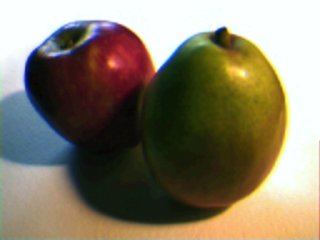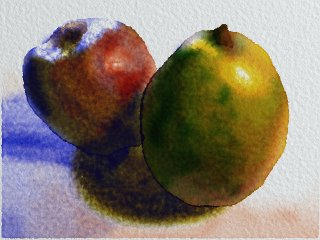A A' B B'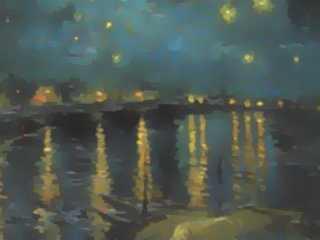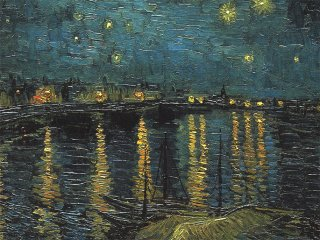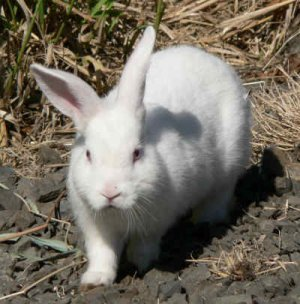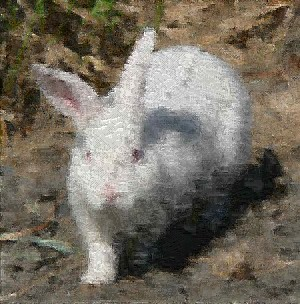A A' B B'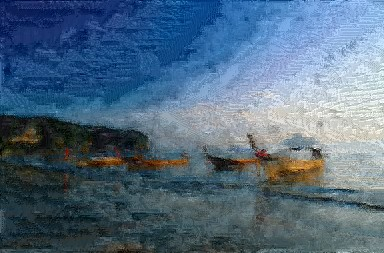A A' B B'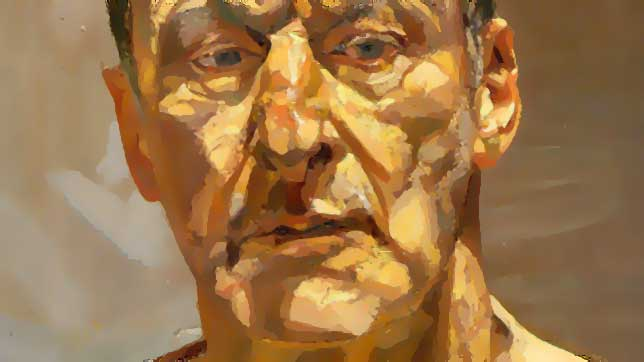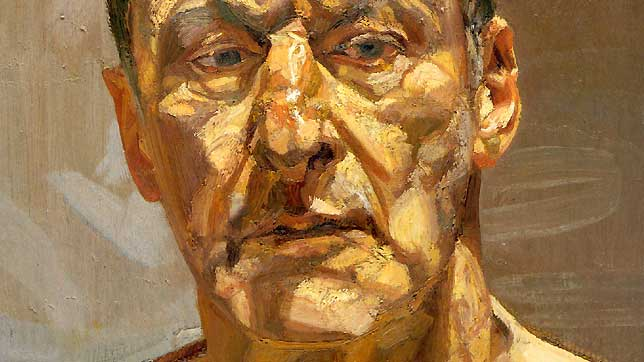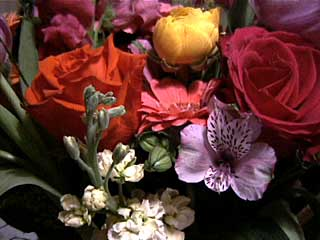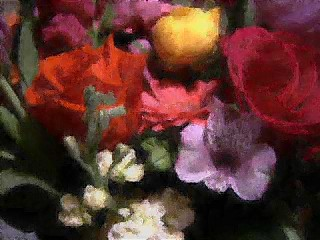A A' B B'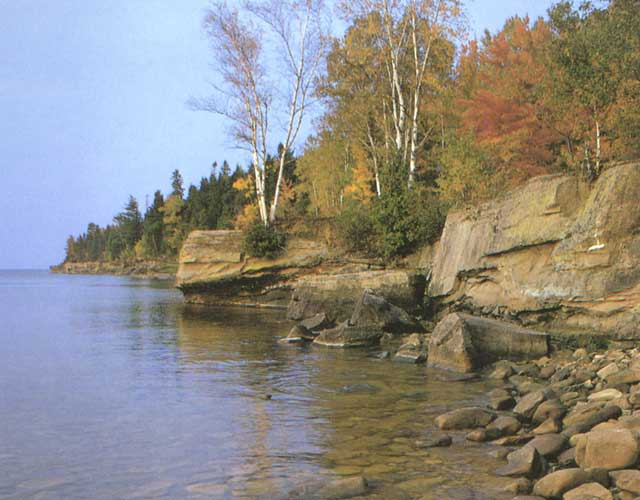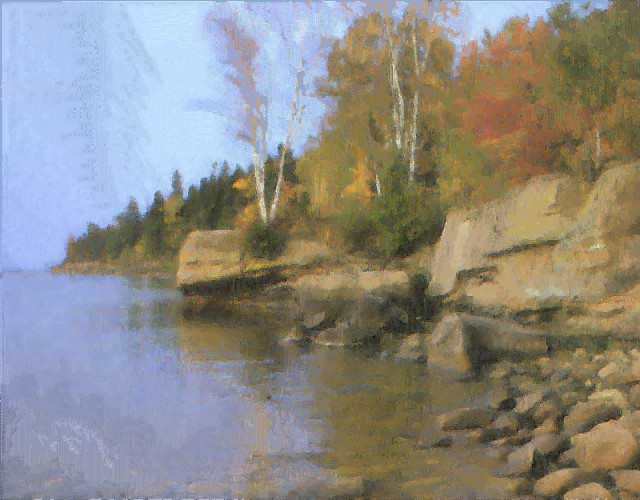#### b. Basic Filters:

 A A' B B'A A' B B'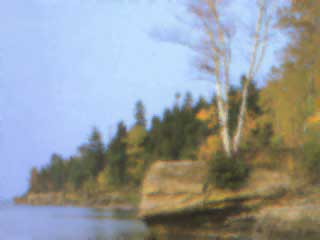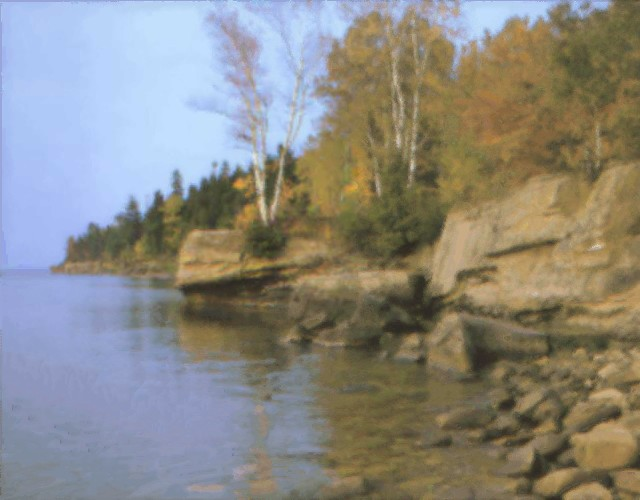#### c. Texture by Number:

The algorithm does a reasonable job here, but the details are not preserved.

 A A' B B'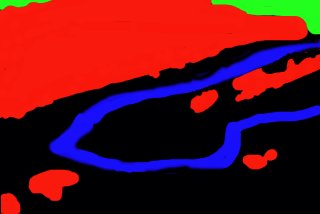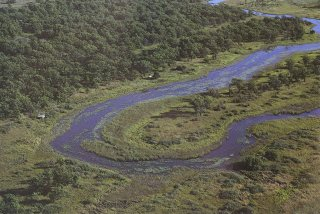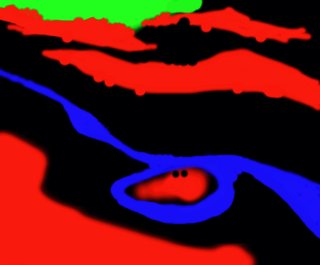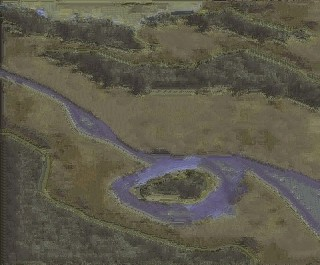A A' B B'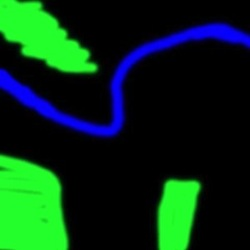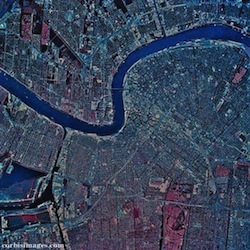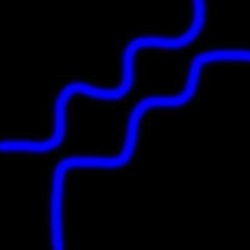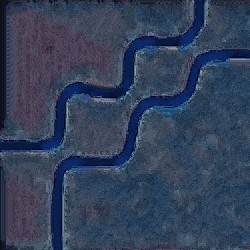A A' B B'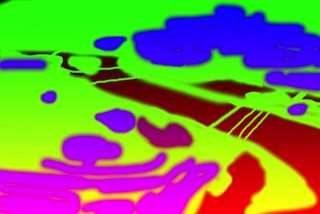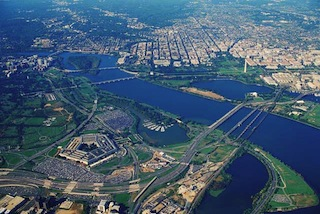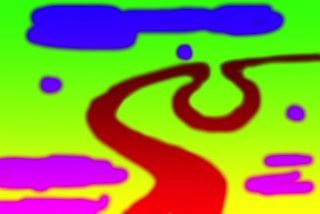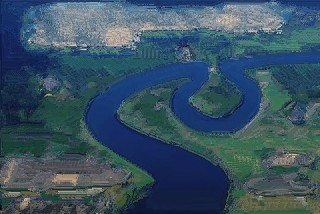A A' B B'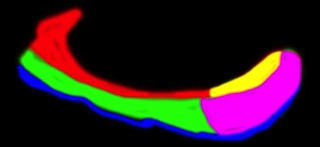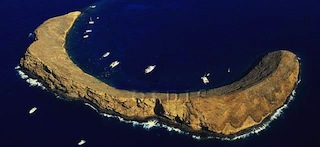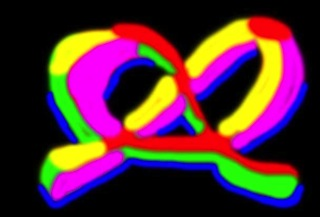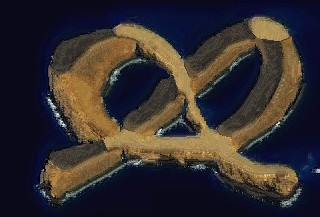A A' B B'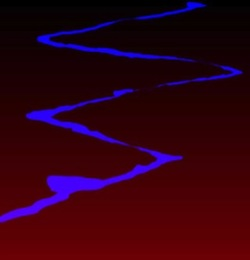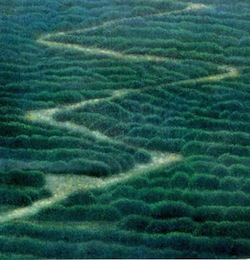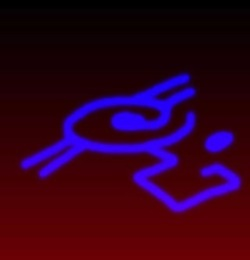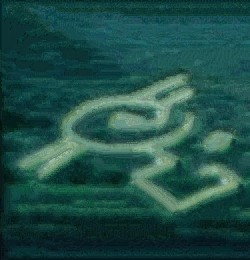#### d. Colorization:

Without using the swatch, the color still looks decent. The second and fifth results are color transfer instead of colorization.

 A B R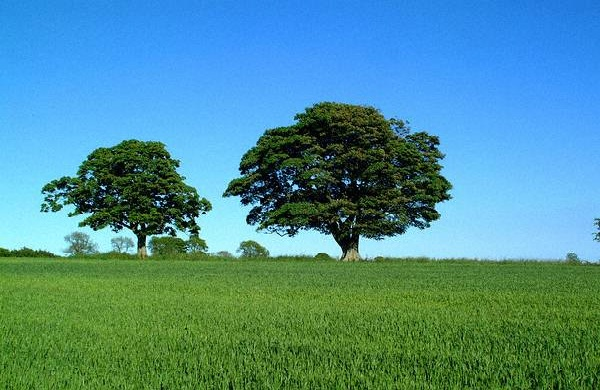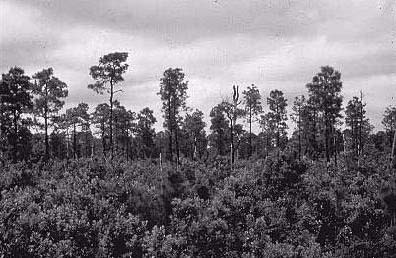A B R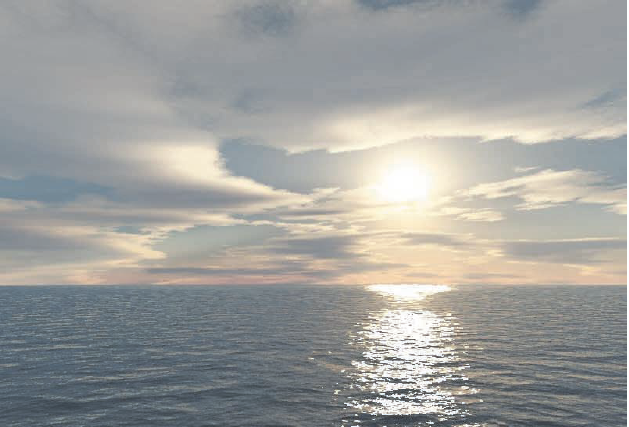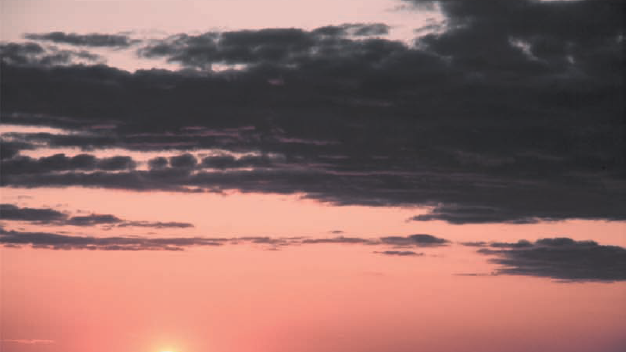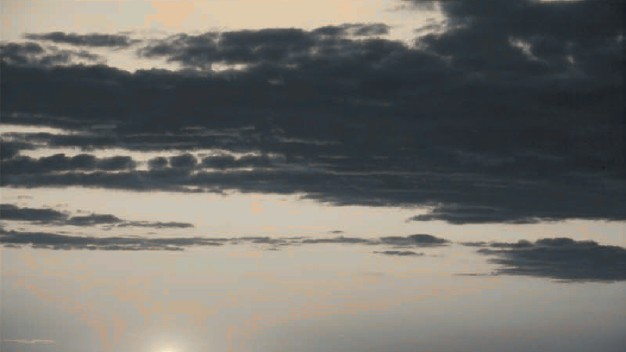A B R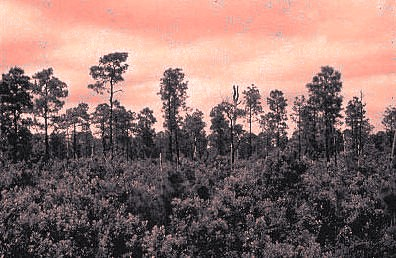A B R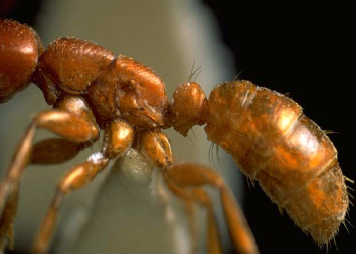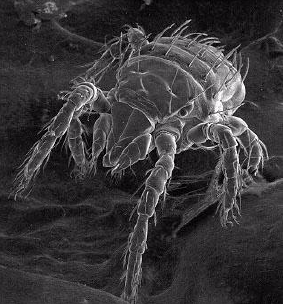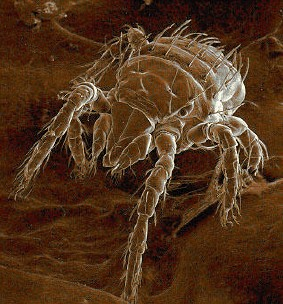A B R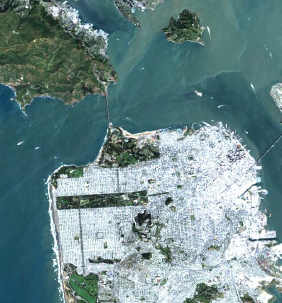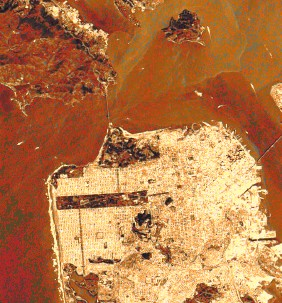A B R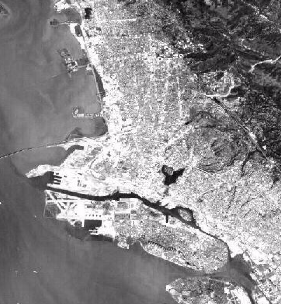#### e. Super Resolution:

 A A' B B'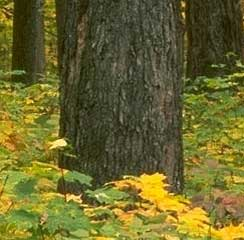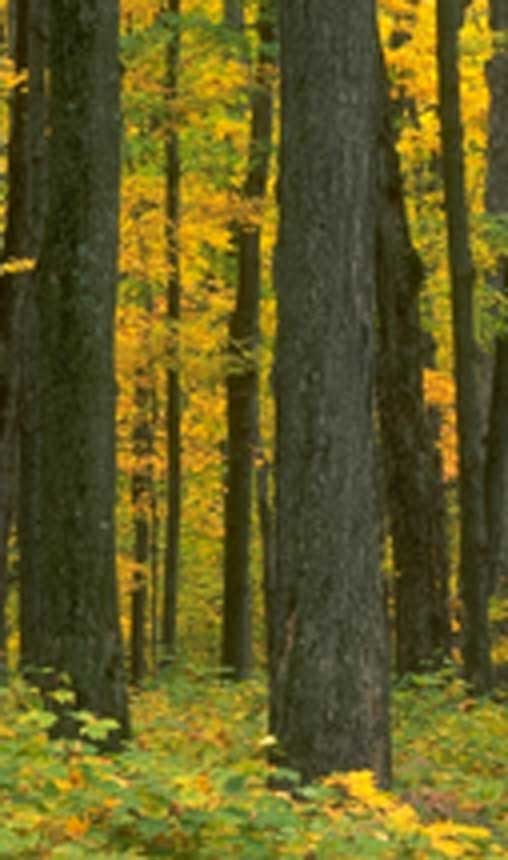A A' B B'